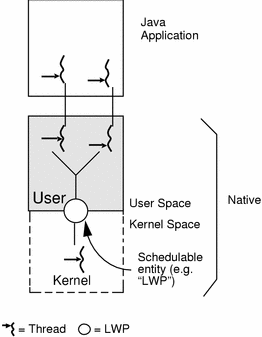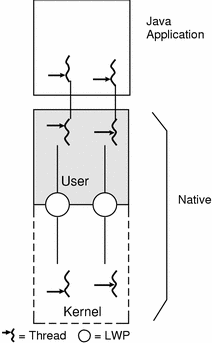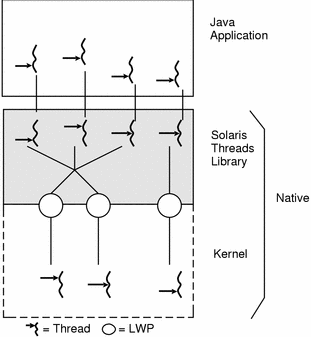JDK 1.1 for Solaris Developer's Guide

Most multithreading models fall into one of the following categories of threading implementation:

• Many-to-One

• One-to-One

• Many-to-Many

Implementations of the many-to-one model (many user threads to one kernel thread) allow the application to create any number of threads that can execute concurrently. In a many-to-one (user-level threads) implementation, all threads activity is restricted to user space. Additionally, only one thread at a time can access the kernel, so only one schedulable entity is known to the operating system. As a result, this multithreading model provides limited concurrency and does not exploit multiprocessors. The initial implementation of Java threads on the Solaris system was many-to-one, as shown in the following figure.

##### Figure 2-1 Many-to-One Multithreading Model## One-to-One Model

##### Figure 2-2 One-to-One Multithreading Model## Many-to-Many Model (Java on Solaris--Native Threads)

The many-to-many model (many user-level threads to many kernel-level threads) avoids many of the limitations of the one-to-one model, while extending multithreading capabilities even further. The many-to-many model, also called the two-level model, minimizes programming effort while reducing the cost and weight of each thread.

In the many-to-many model, a program can have as many threads as are appropriate without making the process too heavy or burdensome. In this model, a user-level threads library provides sophisticated scheduling of user-level threads above kernel threads. The kernel needs to manage only the threads that are currently active. A many-to-many implementation at the user level reduces programming effort as it lifts restrictions on the number of threads that can be effectively used in an application.

A many-to-many multithreading implementation thus provides a standard interface, a simpler programming model, and optimal performance for each process. The Java on Solaris operating environment is the first many-to-many commercial implementation of Java on an MT operating system.

##### Figure 2-3 Many-to-Many Multithreading Model